# 序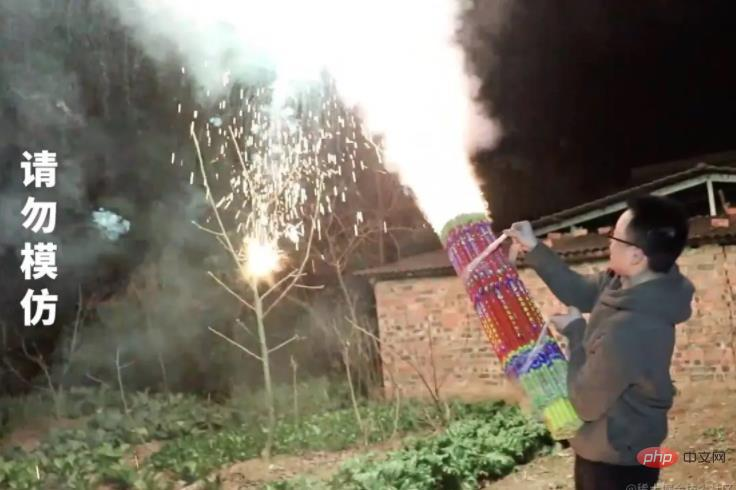2023 过春年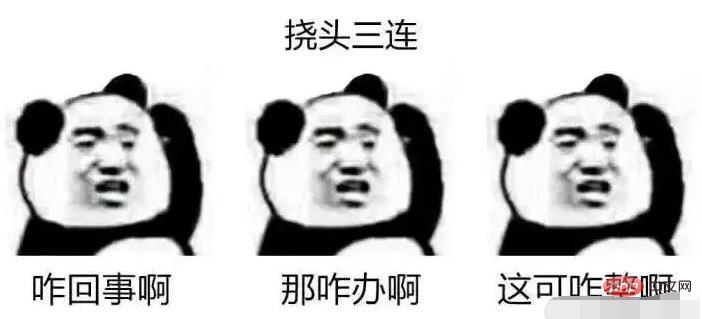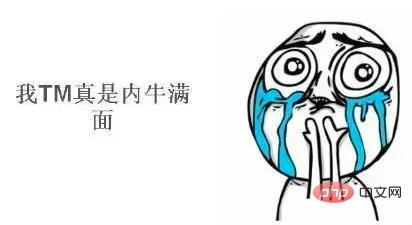2023 过春年，

# 代码

• html：用来构建项目结构

• css：处理文字样式

• js（核心）：处理烟花逻辑

## 1. html

1. 文字结构：用来处理右下角的文本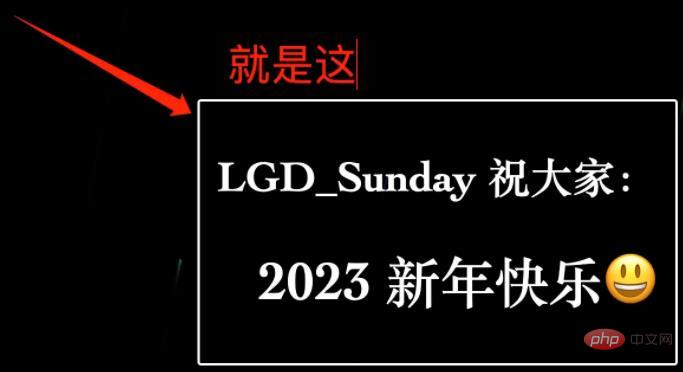2. canvas 画板：作为烟花渲染区

### 1. 文字结构

```<!-- 文字修改区 --> <div class="title"> <h2>LGD_Sunday 祝大家：</h2> <h1>2023 新年快乐?</h1> </div>登录后复制```

### 2. canvas

canvas 作为 `web` 为我们提供的画板工具，可以帮助咱们绘制各种各样的图形。

```<!-- 烟花渲染区 --> <canvas></canvas>登录后复制```

### html 区域总结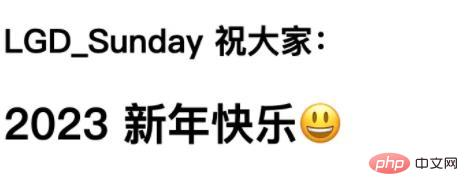## 2. css

`css` 处理的核心目的，是为了帮助咱们绘制文字区域的样式（没错，与烟花无关）。

```html, body { padding: 0px; margin: 0px; background: #222; font-family: 'Karla', sans-serif; color: #fff; height: 100vh; overflow: hidden; } .title { z-index: 1000; position: fixed; bottom: 12px; right: 12px; // 此处修改了字体 font-family: Cambria, Cochin, Georgia, Times, 'Times New Roman', serif; border: 2px solid #fff; padding: 7.5px 15px; background: rgba(0, 0, 0, 0.5); border-radius: 3px; overflow: hidden; } h1 { text-align: right; font-size: 46px; } h2 { font-size: 36px; } canvas { width: 100%; height: 100%; }登录后复制```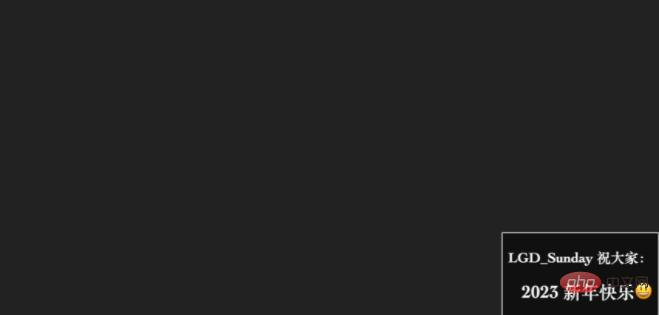## 3. 核心区域：JavaScript

1. 烟花类 Firework：这里咱们会通过`function`构建构造函数，创建出自底向上烟花升起效果。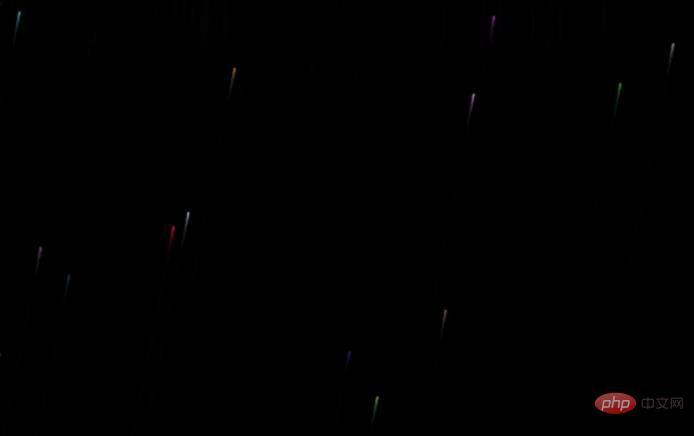2. 火花类 Spark：烟花上升到一定位置，会“绽放“，绽放之后变为”火花“，火花类就是处理绽放之后的火花效果的。1. 渲染函数 render：想要完成整个绽放，需要对页面进行不断地重绘，否则”动画“会卡在一个点不动。所以此时就需要借助到渲染函数，咱们把它叫做 `render`（vue 代码对我影响颇深啊）

2. 工具函数：工具函数主要包含两个，咱们分开去说：

1. 持续绘制函数 drawCircle：该函数可以帮助我们，在每次重新渲染时，进行烟花和火花的绘制
2. 随机色值函数 randomColor：该函数可以帮助我们，得到一个随机的烟花色值，用来生成新的烟花

### 1. 工具函数

```// 获取 canvas 上下文，并指定宽高 let ctx = document.querySelector('canvas').getContext('2d') ctx.canvas.width = window.innerWidth ctx.canvas.height = window.innerHeight /** * 持续绘制 */ function drawCircle(x, y, radius, color) { color = color ctx.fillStyle = color ctx.fillRect(x - radius / 2, y - radius / 2, radius, radius) }登录后复制```

1. 首先：我们拿到了`ctx`，也就是 `CanvasRenderingContext2D` 二维渲染上下文 ，利用它可以进行绘制渲染
2. 然后：把`canvas`的宽高指定为页面宽高
3. 最后：构建了`drawCircle`函数，进行持续绘制。它接收四个参数：
1. `x`：绘制的 x 坐标
2. `y`：绘制的 y 坐标
3. `radius`：点的直径（宽高）
4. `color`：色值

```/** * 生成随机色值 */ function randomColor() { const r = Math.floor(Math.random() * 255) const g = Math.floor(Math.random() * 255) const b = Math.floor(Math.random() * 255) return `rgb(\${r},\${g},\${b})` }登录后复制```

`randomColor` 函数，主要利用`Math.random` 生成了一个 `0-255` 的随机数，三个随机数共同组成了`rgb` 色值。

### 2. 烟花类 Firework

```/** * 烟花构造 */ function Firework(x, y) { // 初始点 this.x = x || Math.random() * ctx.canvas.width this.y = y || ctx.canvas.height // 绽放点 this.burstLocation = (Math.random() * ctx.canvas.height) / 2 // 绽放是否已完毕 this.over = false // 烟花色 this.color = randomColor() }登录后复制```

1. `x、y`：表示烟花升起的坐标。其中`y`中默认为浏览器底部`x`则可以随机
2. `burstLocation`：表示定义的绽放点。通常小于屏幕高度的一半，即：在屏幕上半部分”绽放“
3. `over`：表示当前实例对象是否已经绽放完毕了。完毕的实例将不再处理
4. `color`：随机得到的烟花色

```// 初始绽放数 const OVERLAP_NUM = 66 // 刷新速度 ms const TIME_STEP = 16 // 烟花移动的速度与方向控制 const WALK = 0.2 // 火花数组 let sparks = [] // 烟花数组 let fireworks = [] /** * 烟花构造 */ function Firework(x, y) { ... /** * 移动的方法 */ this.move = function () { // 横向偏移 this.x += WALK // 上升与绽放 if (this.y > this.burstLocation) { this.y -= 1 } else { this.burst() } } /** * 持续绘制 */ this.draw = function () { drawCircle(this.x, this.y, 1.5, this.color) } /** * 绽放方法 */ this.burst = function () { // 标记绽放完毕 this.over = true // 碎裂烟花数 let i = Math.floor(Math.random() * 150) + 10 // 构建碎裂对象 while (i--) { sparks.push(new Spark(this.x, this.y, this.color)) } } }登录后复制```

1. `move`：用来处理烟花上升。在上升的过程中，可以通过`this.x += WALK`来进行横向的”微调“，这样会更加漂亮
2. `draw`：用来进行持续绘制。主要借助了`drawCircle`完成
3. `burst`：处理绽放。当烟花上升到指定位置时，就需要进行绽放处理。所有的绽放过程，将交由`Spark`类处理。

### 3. 火花类 Spark

```/** * 火花构造 */ function Spark(x, y, color) { // 标记绽放点位置与色值 this.x = x this.y = y this.color = color // 位置 this.dir = Math.random() * (Math.PI * 2) // 执行完毕 this.over = false // 火花崩裂速度 this.speed = Math.random() * 3 + 3 // 火花下坠的速度 this.gravity = Math.random() + 0.1 // 火花消失的速度 this.countdown = this.speed * 10 }登录后复制```

1. `x、y、color`：表示绽放的位置与色值
2. `dir`：表示绽放后的位置
3. `over`：表示绽放完成
4. `speed`：火花崩裂之后的运行速度
5. `gravity`：火花开始下坠时的速度，它在计算时会进行递增（加速）
6. `countdown`：消失的倒计时

```/** * 火花构造 */ function Spark(x, y, color) { ... /** * 火花移动方法 */ this.move = function () { // 倒计时处理 this.countdown-- if (this.countdown < 0) { this.over = true } // 速度递减 if (this.speed > 0) { this.speed -= 0.1 } if (this.speed < 0) { return } // x、y 坐标位置 this.x += Math.cos(this.dir + WALK) * this.speed this.y += Math.sin(this.dir + WALK) * this.speed this.y += this.gravity // 下坠速度加快 this.gravity += 0.05 } /** * 绘制 */ this.draw = function () { drawCircle(this.x, this.y, 3, this.color) } }登录后复制```

1. `move`：代表移动的过程。在这里咱们利用Math.cosMath.sin 计算了坐标位置，并且通过`this.gravity += 0.05` 增加了烟花下坠的速度。
2. `draw`：代表持续绘制。同样需要利用`drawCircle`方法。

### 4. 渲染函数 render

`render` 函数的核心作用是：保证烟花的不断渲染。要达到这个目的，咱们就必须要保证`render 函数不断重复执行`

1.window.requestAnimationFrame：该方法可以保证高性能的持续重绘。但是高刷屏幕下会导致 ”速率过快“2.window.setTimeout：该方法可以通过`delay`控制速率，所以在当前场景中比较推荐。

```/** * 渲染函数 */ function render() { // 夜幕背景色与区域 ctx.fillStyle = 'rgba(0, 0, 0, 0.1)' ctx.fillRect(0, 0, ctx.canvas.width, ctx.canvas.height) // 烟花上升 for (let firework of fireworks) { if (firework.over) { continue } firework.move() firework.draw() } // 火花下坠 for (let spark of sparks) { if (spark.over) { continue } spark.move() spark.draw() } // 通过随机数来控制烟花产生速度 if (Math.random() < 0.05) { fireworks.push(new Firework()) } // 重复渲染 setTimeout(render, TIME_STEP) }登录后复制```

### 5. 完整代码

```// 获取 canvas 上下文，并指定宽高 let ctx = document.querySelector('canvas').getContext('2d') ctx.canvas.width = window.innerWidth ctx.canvas.height = window.innerHeight // 初始绽放数 const OVERLAP_NUM = 66 // 刷新速度 ms const TIME_STEP = 16 // 烟花移动的速度与方向控制 const WALK = 0.2 // 火花数组 let sparks = [] // 烟花数组 let fireworks = [] // 初始爆炸的填充逻辑 for (let i = 0; i < OVERLAP_NUM; i++) { // 填充 fireworks.push( // 构建随机位置 new Firework( Math.random() * window.innerWidth, Math.random() * window.innerHeight ) ) } /** * 渲染函数 */ function render() { // 夜幕背景色与区域 ctx.fillStyle = 'rgba(0, 0, 0, 0.1)' ctx.fillRect(0, 0, ctx.canvas.width, ctx.canvas.height) // 烟花上升 for (let firework of fireworks) { if (firework.over) { continue } firework.move() firework.draw() } // 火花下坠 for (let spark of sparks) { if (spark.over) { continue } spark.move() spark.draw() } // 通过随机数来控制烟花产生速度 if (Math.random() < 0.05) { fireworks.push(new Firework()) } // 重复渲染 setTimeout(render, TIME_STEP) } /** * 火花构造 */ function Spark(x, y, color) { // 标记绽放点位置与色值 this.x = x this.y = y this.color = color // 位置 this.dir = Math.random() * (Math.PI * 2) // 执行完毕 this.over = false // 火花崩裂速度 this.speed = Math.random() * 3 + 3 // 火花下坠的速度 this.gravity = Math.random() + 0.1 // 火花消失的速度 this.countdown = this.speed * 10 /** * 火花移动方法 */ this.move = function () { // 倒计时处理 this.countdown-- if (this.countdown < 0) { this.over = true } // 速度递减 if (this.speed > 0) { this.speed -= 0.1 } if (this.speed < 0) { return } // x、y 坐标位置 this.x += Math.cos(this.dir + WALK) * this.speed this.y += Math.sin(this.dir + WALK) * this.speed this.y += this.gravity // 下坠速度加快 this.gravity += 0.05 } /** * 绘制 */ this.draw = function () { drawCircle(this.x, this.y, 3, this.color) } } /** * 烟花构造 */ function Firework(x, y) { // 初始点 this.x = x || Math.random() * ctx.canvas.width this.y = y || ctx.canvas.height // 绽放点 this.burstLocation = (Math.random() * ctx.canvas.height) / 2 // 绽放是否已完毕 this.over = false // 烟花色 this.color = randomColor() /** * 移动的方法 */ this.move = function () { // 横向偏移 this.x += WALK // 上升与绽放 if (this.y > this.burstLocation) { this.y -= 1 } else { this.burst() } } /** * 持续绘制 */ this.draw = function () { drawCircle(this.x, this.y, 1.5, this.color) } /** * 绽放方法 */ this.burst = function () { // 标记绽放完毕 this.over = true // 碎裂烟花数 let i = Math.floor(Math.random() * 150) + 10 // 构建碎裂对象 while (i--) { sparks.push(new Spark(this.x, this.y, this.color)) } } } /** * 持续绘制 */ function drawCircle(x, y, radius, color) { color = color ctx.fillStyle = color ctx.fillRect(x - radius / 2, y - radius / 2, radius, radius) } /** * 生成随机色值 */ function randomColor() { const r = Math.floor(Math.random() * 255) const g = Math.floor(Math.random() * 255) const b = Math.floor(Math.random() * 255) return `rgb(\${r},\${g},\${b})` } // 开始 render()登录后复制```

# 总结

2023 年将会是一个好的年度，大家一起加油！

• 相关标签：前端 JavaScript 程序员
• 相关文章

相关视频